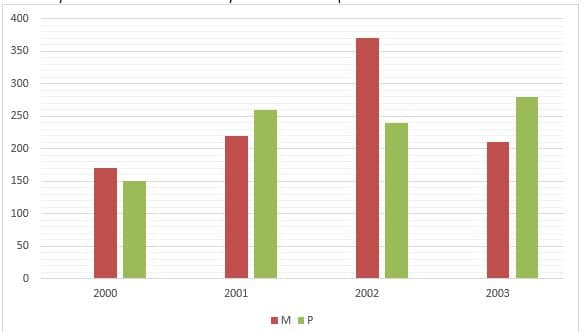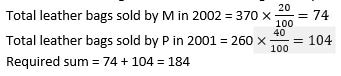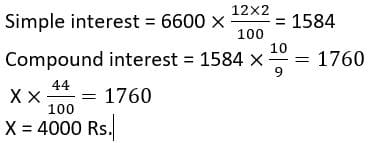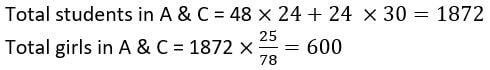# SBI PO Prelims Memory Based Paper 2019 : Questions asked in all shifts

## SBI PO Prelims Memory Based Paper 2019:

The most awaited exam of the year, SBI PO Pre 2019, has started from 8th June with in 4 shifts. and the SBI PO Pre exam 1st shift is over now. so here we are come out with the memory based paper of SBI PO Pre 2019 or you can say Questions asked in SBI PO Prelims exam 8th June 2019. Check below the questions asked in SBI PO Pre Exam today. Download SBI PO PRE Memory Based Paper from the mentioned below link:

### English Language SBI PO PRE Memory Based Paper

Quantitative Aptitude SBI PO PRE Memory Based Paper

### Questions Asked in 4th Shift 8th June 2019 (Quantitative Aptitude)

Direction (1 – 5): Bar graph given below shows number of bangs sold by two companies (M & P) in four different years. Read the data carefully and answer the questions.Q1. Out of total bags sold by M in 2002, 20% are leather bags and out of total bags sold by P in 2001, 40% are leather bags. Find total leather bags sold by P & M together?Q2. If average number of bags sold by M in 2002, 2003 & 2004 is 310, then find total number of bags sold by M in the years 2004?

Total bags sold by M in the years 2004  = 310 * 3 – (370+210) = 350

Q3. Total bags sold by M in 2000 & 2003 together is what percent more than total bags sold by P in 2000?Q4. Find the ratio of total bags sold by M & P in 2000 to total bags sold by P in 2002 & 2003 together ?

Total bags sold by M & P in 2000 = 170 + 150 = 320

Total bags sold by P in 2002 & 2003 = 240 + 280 = 520

Required ratio = 320 : 520 = 8 : 13

Q5. Total bags sold by M & P together in 2003 is how much more than total bags sold by these two companies in 2001?

Total bags sold by M & P in the years 2003 = 280 + 210 = 490

Total bags sold by M & P in the years 2001 = 220 + 260 = 480

Required difference = 490-480=10

Q6.  A man invested Rs. 6600 on SI for two years at the rate of 12 p.a and Rs. X on CI at the rate of 20% p.a. for two years. If ratio of SI to CI get by man after two years is 9 : 10, then find ‘X’?Q7. Cost price of each of article A & B is Rs. 220. A sold on profit of 16.8 % and B sold on profit of 20.2%. Find the total profit?Q8. A shopkeeper allows 10% discount on an article and get a profit of 12.5%, If another person X sold same article on 40% discount, then find X loss %?Q9. What will be marks of Mohan in math.

1. Marks of Mohan in math is 40% more than that of in English.
2. Average marks of Mohan in math & History is 81 and average marks in English & History is 62.

So, help of I & II together we can find the marks of Mohan in Math.

Q10. What will  be cost price of article ?

I . Difference between cost price & mark price of article is Rs. 240.

II . Shopkeeper made a profit of 25% after selling the article at 20% discount.

So, help of I & II together we can find cost price of article.

Q11. What will be area of circle .

I . Sum of circumference of circle & diameter of circle is equal to perimeter of square which side is 29 cm.

II. Difference of circumference of circle & diameter of circle is 60 cm.

So, either from I or II we can find the area of circle.

Direction (12 – 14): Table below shows number of teachers in five different schools and ratio of teacher to students in these schools.

 Schools Total teacher Ratio of teachers : students A 48 1 : 24 B 36 1 : 28 C 24 I : 30 D 42 3 : 44 E 28 1 : 36

Total people in schools = Teachers + Students + none teaching staff

Q12. If ratio of boys to girl is 53 : 25 in total students in A & C, then find total girls in A & C together?Q13. If total people in B and E are 2384 and difference between total none teaching staff of B & E is 104, then find total none teaching staff in B & E?Q14.  Total students in D is what percent more than total students in E.• Number Series Missing number series questions were asked. The questions were easy. Following number series questions were asked –
1. 7, 9, 18, 57, 232, 1165, 7033= Worng no is 9 (*1+1, *2+2, *3+3…so on)
2. 70, 80, 76, 86, 80, 92, 88= Wrong number is 80 (+6, +6 pattern)
3. 25, 35, 40, 60, 100, 180, 340= Wrong no. 35 (+5, +10, +20, +40, +80, +160)
4. 8, 6, 4, 6, 12, 30, 90= Wrong no. 6 (*0.5, *1, *1.5, *2, * 2.5, *3)

Note – Two more questions asked in this table DI which is calculative.

Tips for next sifts – One di is easy so select it and do it fast as you can, in second di 2 to 3 questions are calculative, so if you are good in calculation go for it, otherwise attempt time & work, SI & Ci these topic these are doable.